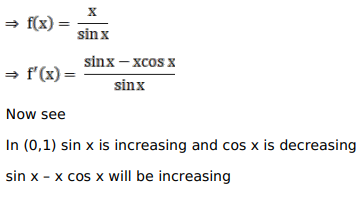# Solve this following

Question:

Mark $(\sqrt{)}$ against the correct answer in the following:

$f(x)=\frac{x}{\sin x}$ is

A. increasing in $(0,1)$

B. decreasing in $(0,1)$

C. increasing in $\left(0, \frac{1}{2}\right)$ and decreasing in $\left(\frac{1}{2}, 1\right)$

D. none of these

Solution:$\therefore f(x)$ is increasing in $(0,1)$